https://doi.org/10.5194/esurf-11-961-2023
https://doi.org/10.5194/esurf-11-961-2023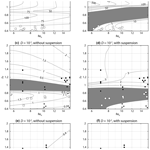# Linear-stability analysis of plane beds under flows with suspended loads

Koji Ohata, Hajime Naruse, and Norihiro Izumi
Abstract

Plane beds develop under flows in fluvial and marine environments; they are recorded as parallel lamination in sandstone beds, such as those found in turbidites. However, whereas turbidites typically exhibit parallel lamination, they rarely feature dune-scale cross-lamination. Although the reason for the scarcity of dune-scale cross-lamination in turbidites is still debated, the formation of dunes may be dampened by suspended loads. Here, we perform, for the first time, linear-stability analysis to show that flows with suspended loads facilitate the formation of plane beds. For a fine-grained bed, a suspended load can promote the formation of plane beds and dampen the formation of dunes. These results of theoretical analysis were verified with observational data of plane beds under open-channel flows. Our theoretical analysis found that suspended loads promote the formation of plane beds, which suggests that the development of dunes under turbidity currents is suppressed by the presence of suspended loads.

Share
Dates
1 Introduction

The interactions between fluids and erodible surfaces generate small-scale topographic features called bedforms on both terrestrial surfaces (e.g., riverbeds, deserts, and deep-sea floors) and extra-terrestrial surfaces . Such bedforms are preserved in sedimentary rocks as sedimentary structures such as cross-lamination and parallel lamination (Harms1979). The types of sedimentary structures observed vary among different types of rocks. Turbidites typically exhibit parallel lamination (Bouma1962), whereas they rarely feature dune-scale cross-lamination . However, the opposite is true for fluvial deposits; i.e., dune-scale cross-laminae are often observed in riverine sandstone (Miall2010).

Although the reason for the paucity of dune-scale cross-lamination in turbidites is still debated , it could be attributed to the presence of suspended loads. For example, in the case of open-channel flows, nearly flat-bed waves and low-angle dunes have been observed in suspension-dominated rivers . Additionally, flume experiments have suggested that dune height decreases with increasing suspended-load flux . Therefore, the influence of suspended loads on the suppression of dune development and the formation of plane beds is worth investigating.

The relationships between sediment transport modes and the formation of plane beds have received little attention in theoretical works that performed linear-stability analyses. The reason could be that previous studies have succeeded in predicting the wavelength of dunes and antidunes without considering suspended loads . However, this assumption is not appropriate for analyzing open-channel flows where the suspended load is not negligible, such as flows in rivers with a fine sediment bed . Moreover, although some research has considered both bed and suspended loads , the hydraulic conditions of these analyses were limited, and the results were tested using only observational data of dunes and antidunes.

Therefore, in order to investigate the effect of sediment transport mode on the formation of plane beds, we performed a linear-stability analysis of bedforms under open-channel flows carrying suspended loads. The model introduced in was extended in this study to evaluate plane bed formation under various conditions of sediment diameter and flow depth. To evaluate the suspended-load effect, linear-stability analyses were performed on flows both with and without suspended loads. Further, we tested our stability diagrams against observational data of plane beds. Our theoretical analysis reveals for the first time that suspended loads promote the formation of plane beds, which has implications for interpreting sedimentary structures in turbidites.

2 Methods

Linear-stability analysis of fluvial bedforms can provide the wavelengths of perturbations (i.e., bed waves) that grow over time . We employ the two-dimensional Reynolds-averaged Navier–Stokes equations as the governing equations for flows and the quasi-steady assumption to neglect the unsteady terms in the flow equations. The eddy viscosity is evaluated using a mixing-length approach. In this study, bed-load discharge is estimated using the Meyer-Peter and Müller formula modified as described in . The entrainment rate of the suspended load is estimated using the relationship proposed in . See the following section for details. To test the results of linear-stability analyses against the observational data of plane beds, we plotted stability diagrams in the parametric space of hydraulic parameters.

## 2.1 Governing parameters

The instability of a system is illustrated as a contour diagram of the perturbation growth rate ωi (Fig. 1). Generally, theoretical studies of bedforms based on linear-stability analyses describe the transition of bedform phases in the parametric space of wavenumber k and Froude number Fr, which are given by

$\begin{array}{}\text{(1)}& k=\frac{\mathrm{2}\mathit{\pi }{\stackrel{\mathrm{̃}}{h}}_{\mathrm{0}}}{\stackrel{\mathrm{̃}}{\mathit{\lambda }}}\text{(2)}& \mathit{Fr}=\frac{{\stackrel{\mathrm{̃}}{U}}_{\mathrm{0}}}{\sqrt{\stackrel{\mathrm{̃}}{g}{\stackrel{\mathrm{̃}}{h}}_{\mathrm{0}}}},\end{array}$

where $\stackrel{\mathrm{̃}}{\mathit{\lambda }}$ denotes the perturbation wavelength, ${\stackrel{\mathrm{̃}}{U}}_{\mathrm{0}}$ is the depth-averaged flow velocity of the uniform flow, $\stackrel{\mathrm{̃}}{g}$ is the gravitational acceleration (i.e., 9.81 m2 s−1), and ${\stackrel{\mathrm{̃}}{h}}_{\mathrm{0}}$ is the flow depth of the uniform flow. Hereafter, we denote dimensional variables using a tilde.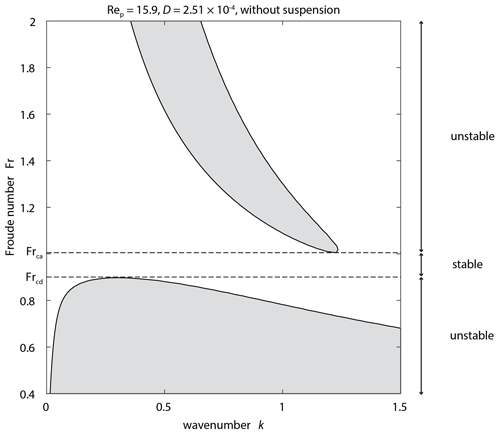Figure 1Contour map of perturbation growth rate ωi without suspension. The particle Reynolds number and dimensionless particle diameter were set to Rep=15.9 and $D=\mathrm{2.51}×{\mathrm{10}}^{-\mathrm{4}}$, respectively. The dotted line denotes the threshold of sediment motion. The dashed lines denote the critical Froude numbers Frcd and Frca for instabilities. The region where the growth rate is positive is highlighted in gray.

Stability diagrams described on the kFr plane have been commonly used to predict the development of dunes and antidunes (Kennedy1963). A few studies have used other combinations of dimensionless numbers such as the friction coefficient C versus Fr and the relative roughness $\stackrel{\mathrm{̃}}{D}/{\stackrel{\mathrm{̃}}{h}}_{\mathrm{0}}$ on the kFr plane .

Although the classic kFr diagrams are widely accepted, we cannot use this approach to evaluate whether plane bed formation can be predicted reliably because plane beds have extremely small wavenumbers or have an infinite wavelength (i.e., they are flat). Therefore, we illustrate stability diagrams as contour maps of the maximum growth rate ωi,max of the instability on the RepFr plane with fixed D and on the DFr plane with fixed Rep to investigate the impact of suspended loads on the formation of plane beds. Here, D denotes the dimensionless particle diameter $\stackrel{\mathrm{̃}}{D}/{\stackrel{\mathrm{̃}}{h}}_{\mathrm{0}}$.

The instability of a system is illustrated as a contour diagram of the perturbation growth rate ωi (Fig. 1). We can rewrite Eq. (A30) as

$\begin{array}{}\text{(3)}& \mathit{\omega }=\mathit{\omega }\left(k,Fr,\stackrel{\mathrm{̃}}{D},{\stackrel{\mathrm{̃}}{h}}_{\mathrm{0}}\right).\end{array}$

Thus, we can obtain the growth rate ωi as a function of k for a given combination of Fr, $\stackrel{\mathrm{̃}}{D}$, and ${\stackrel{\mathrm{̃}}{h}}_{\mathrm{0}}$ (Figs. A3 and A4; Tables A1–A5). In this study, we assume that the system is stable if ωi is not positive for all k within the domain [kmin, kmax] for a given Fr, $\stackrel{\mathrm{̃}}{D}$, and ${\stackrel{\mathrm{̃}}{h}}_{\mathrm{0}}$ combination. In contrast, the system is assumed to be unstable if ωi is positive for some k (Fig. 1). We describe stability diagrams as contour maps of the maximum growth rate of the instability in the parametric space of (Rep,Fr) (Fig. 2) and (D,Fr) (Fig. 3).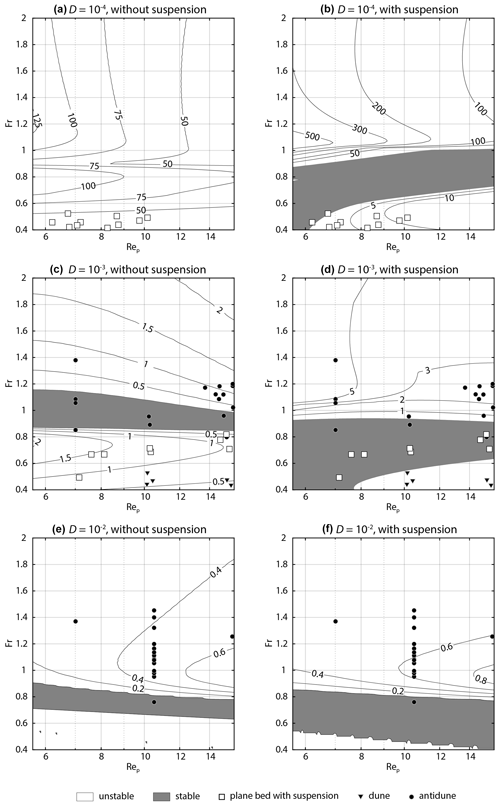Figure 2Contour maps of the maximum growth rate ωi,max of perturbations with a fixed dimensionless particle diameter D. Symbols are observational data. (a) $D={\mathrm{10}}^{-\mathrm{4}}$ without suspension. (b) $D={\mathrm{10}}^{-\mathrm{4}}$ with suspension. (c) $D={\mathrm{10}}^{-\mathrm{3}}$ without suspension. (d) $D={\mathrm{10}}^{-\mathrm{3}}$ with suspension. (e) $D={\mathrm{10}}^{-\mathrm{2}}$ without suspension. (f) $D={\mathrm{10}}^{-\mathrm{2}}$ with suspension. (a, b) The range of D of observational data is from $\mathrm{3.16}×{\mathrm{10}}^{-\mathrm{5}}$ to $\mathrm{3.16}×{\mathrm{10}}^{-\mathrm{4}}$. (c, d) The range of D of observational data is from $\mathrm{3.16}×{\mathrm{10}}^{-\mathrm{4}}$ to $\mathrm{3.16}×{\mathrm{10}}^{-\mathrm{3}}$. (e, f) The range of D of observational data is from $\mathrm{3.16}×{\mathrm{10}}^{-\mathrm{3}}$ to $\mathrm{3.16}×{\mathrm{10}}^{-\mathrm{2}}$.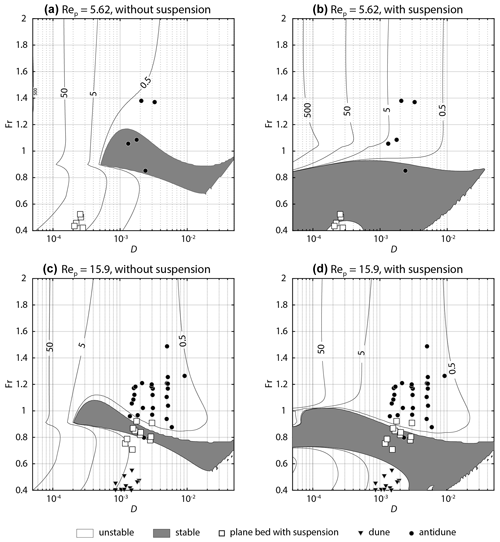Figure 3Contour maps of the maximum growth rate ωi,max of perturbations with a fixed particle Reynolds number Rep. Symbols are observational data. (a) Rep=5.62 without suspension. (b) Rep=5.62 with suspension. (c) Rep=15.9 without suspension. (d) Rep=15.9 with suspension. (a, b) The range of Rep of observational data is from 4.46 to 7.0749. (c, d) The range of Rep of observational data is from 12.6 to 20.

The domain [kmin,kmax] was set as [0.01,1.5], corresponding to λ ranging from ∼4.2h to ∼628h. The Froude number range was set as 0.42. For the RepFr diagram (Fig. 2), we employed three grades of D: $D={\mathrm{10}}^{-\mathrm{4}},{\mathrm{10}}^{-\mathrm{3}}$, and 10−2. The particle Reynolds number Rep ranges from 5.62 to 15.9 ($\stackrel{\mathrm{̃}}{D}=$ 0.125–0.25 mm). For the DFr diagram, we employed Rep=5.62 and 15.9 as the fixed value of particle diameter. The dimensionless particle diameter D ranges from $\mathrm{5.0}×{\mathrm{10}}^{-\mathrm{2}}$ to $\mathrm{5.0}×{\mathrm{10}}^{-\mathrm{5}}$ in the DFr diagram (Fig. 3).

## 2.2 Linear-stability analysis

Here we present the formulation of the problem and the method used to solve the differential equations.

### 2.2.1 Flow equations

The governing equations for flows are the two-dimensional Reynolds-averaged Navier–Stokes equations. On erodible beds, the flow adjustments occur immediately relative to the bed adjustments . Therefore, we employ the quasi-steady assumption to neglect the unsteady terms in the flow equations .

Under the quasi-steady assumption, the dimensionless forms of the Reynolds-averaged Navier–Stokes equations and continuity equation for incompressible flow are described as

$\begin{array}{}\text{(4)}& u\frac{\partial u}{\partial x}+w\frac{\partial u}{\partial z}=-\frac{\partial p}{\partial x}+\mathrm{1}+\frac{\partial {T}_{xx}}{\partial x}+\frac{\partial {T}_{xz}}{\partial z}\text{(5)}& u\frac{\partial w}{\partial x}+w\frac{\partial w}{\partial z}=-\frac{\partial p}{\partial z}+{S}^{-\mathrm{1}}+\frac{\partial {T}_{xz}}{\partial x}+\frac{\partial {T}_{zz}}{\partial z}\text{(6)}& \frac{\partial u}{\partial x}+\frac{\partial w}{\partial z}=\mathrm{0},\end{array}$

where u and w are the flow velocities in the x and z directions, respectively; p denotes the pressure; S is the bed slope; and ${T}_{ij}\left(i,j=x,z\right)$ is the Reynolds stress tensor.

We employ a Boussinesq-type assumption to close the flow equations:

$\begin{array}{}\text{(7)}& {T}_{xx}=\mathrm{2}{\mathit{\nu }}_{T}\frac{\partial u}{\partial x}\text{(8)}& {T}_{zz}=\mathrm{2}{\mathit{\nu }}_{T}\frac{\partial w}{\partial z}\text{(9)}& {T}_{xz}={\mathit{\nu }}_{T}\left(\frac{\partial u}{\partial x}+\frac{\partial w}{\partial z}\right).\end{array}$

Then, the eddy viscosity νT is evaluated using a mixing-length approach:

$\begin{array}{}\text{(10)}& {\mathit{\nu }}_{T}={l}^{\mathrm{2}}\left|\frac{\partial u}{\partial z}\right|\text{(11)}& l=\mathit{\kappa }\left(z-Z\right)\sqrt{\frac{h+R-z}{h}},\end{array}$

where l is the mixing length, κ is the Kármán coefficient (i.e., 0.4), h is the flow depth, Z denotes the bed height, and R is the height of the reference level at which the flow velocity is assumed to vanish in a logarithmic profile (Fig. A1).

In the above equations, the system is non-dimensionalized as follows:

$\begin{array}{}\text{(12)}& \left(u,w\right)=\left(\stackrel{\mathrm{̃}}{u},\stackrel{\mathrm{̃}}{w}\right)/{\stackrel{\mathrm{̃}}{u}}_{{\mathrm{f}}_{\mathrm{0}}}\text{(13)}& \left(x,z,h,Z,R,D\right)=\left(\stackrel{\mathrm{̃}}{x},\stackrel{\mathrm{̃}}{z},\stackrel{\mathrm{̃}}{h},\stackrel{\mathrm{̃}}{Z},\stackrel{\mathrm{̃}}{R},\stackrel{\mathrm{̃}}{D}\right)/{\stackrel{\mathrm{̃}}{h}}_{\mathrm{0}}\text{(14)}& \left(p,{T}_{ij}\right)=\left(\stackrel{\mathrm{̃}}{p},{\stackrel{\mathrm{̃}}{T}}_{ij}\right)/\stackrel{\mathrm{̃}}{\mathit{\rho }}{\stackrel{\mathrm{̃}}{h}}_{\mathrm{0}}\text{(15)}& {\mathit{\nu }}_{T}={\stackrel{\mathrm{̃}}{\stackrel{\mathrm{̃}}{\mathit{\nu }}}}_{T}/\left({\stackrel{\mathrm{̃}}{u}}_{{\mathrm{f}}_{\mathrm{0}}}{\stackrel{\mathrm{̃}}{h}}_{\mathrm{0}}\right),\end{array}$

where D is the non-dimensional diameter of a bed particle, ${\stackrel{\mathrm{̃}}{u}}_{{\mathrm{f}}_{\mathrm{0}}}$ denotes the shear velocity in the basic flat-bed state, and $\stackrel{\mathrm{̃}}{\mathit{\rho }}$ is the water density (i.e., 1000 kg m3). The shear velocity in the basic flat-bed state ${\stackrel{\mathrm{̃}}{u}}_{{\mathrm{f}}_{\mathrm{0}}}$ is obtained as

$\begin{array}{}\text{(16)}& {\stackrel{\mathrm{̃}}{u}}_{{\mathrm{f}}_{\mathrm{0}}}=\sqrt{\stackrel{\mathrm{̃}}{g}{\stackrel{\mathrm{̃}}{h}}_{\mathrm{0}}S}.\end{array}$

As the flow is continuous, the system can be rewritten using the stream function ψ defined as

$\begin{array}{}\text{(17)}& \left(u,w\right)=\left(\frac{\partial \mathit{\psi }}{\partial z},-\frac{\partial \mathit{\psi }}{\partial x}\right).\end{array}$

Then, Eqs. (4) and (5) are rearranged to

$\begin{array}{}\text{(18)}& \begin{array}{rl}\frac{\partial \mathit{\psi }}{\partial z}\frac{{\partial }^{\mathrm{2}}\mathit{\psi }}{\partial x\partial z}-\frac{\partial \mathit{\psi }}{\partial x}\frac{{\partial }^{\mathrm{2}}\mathit{\psi }}{\partial {z}^{\mathrm{2}}}=& -\frac{\partial p}{\partial x}+\mathrm{1}+\frac{\partial }{\partial x}\left(\mathrm{2}{\mathit{\nu }}_{T}\frac{{\partial }^{\mathrm{2}}\mathit{\psi }}{\partial x\partial z}\right)\\ & +\frac{\partial }{\partial z}\left[{\mathit{\nu }}_{T}\left(\frac{{\partial }^{\mathrm{2}}\mathit{\psi }}{\partial {z}^{\mathrm{2}}}\phantom{\rule{0.33em}{0ex}}-\frac{{\partial }^{\mathrm{2}}\mathit{\psi }}{\partial {x}^{\mathrm{2}}}\right)\right]\end{array}\text{(19)}& \begin{array}{rl}\frac{\partial \mathit{\psi }}{\partial x}\frac{{\partial }^{\mathrm{2}}\mathit{\psi }}{\partial x\partial z}-\frac{\partial \mathit{\psi }}{\partial z}\frac{{\partial }^{\mathrm{2}}\mathit{\psi }}{\partial {x}^{\mathrm{2}}}=& -\frac{\partial p}{\partial z}+{S}^{-\mathrm{1}}-\frac{\partial }{\partial z}\left(\mathrm{2}{\mathit{\nu }}_{T}\frac{{\partial }^{\mathrm{2}}\mathit{\psi }}{\partial x\partial z}\right)\\ & +\frac{\partial }{\partial x}\left[{\mathit{\nu }}_{T}\left(\frac{{\partial }^{\mathrm{2}}\mathit{\psi }}{\partial {z}^{\mathrm{2}}}\phantom{\rule{0.33em}{0ex}}-\frac{{\partial }^{\mathrm{2}}\mathit{\psi }}{\partial {x}^{\mathrm{2}}}\right)\right]\end{array}.\end{array}$

Eliminating p from Eqs. (18) and (19), we obtain

$\begin{array}{}\text{(20)}& \begin{array}{rl}\frac{\partial \mathit{\psi }}{\partial z}\frac{\partial }{\partial x}{\mathrm{\nabla }}^{\mathrm{2}}\mathit{\psi }& -\frac{\partial \mathit{\psi }}{\partial x}\frac{\partial }{\partial z}{\mathrm{\nabla }}^{\mathrm{2}}\mathit{\psi }-\mathrm{4}\frac{{\partial }^{\mathrm{2}}}{\partial x\partial z}\left({\mathit{\nu }}_{T}\frac{{\partial }^{\mathrm{2}}\mathit{\psi }}{\partial x\partial z}\right)\\ & +\phantom{\rule{0.125em}{0ex}}\left(\frac{{\partial }^{\mathrm{2}}}{\partial {x}^{\mathrm{2}}}-\frac{{\partial }^{\mathrm{2}}}{\partial {z}^{\mathrm{2}}}\right)\left[{\mathit{\nu }}_{T}\phantom{\rule{0.125em}{0ex}}\left(\frac{{\partial }^{\mathrm{2}}}{\partial {z}^{\mathrm{2}}}-\frac{{\partial }^{\mathrm{2}}}{\partial {x}^{\mathrm{2}}}\right)\phantom{\rule{0.125em}{0ex}}\mathit{\psi }\right]=\mathrm{0}\end{array}.\end{array}$

### 2.2.2 Advection–diffusion equations for suspended sediment

We also assume a quasi-steady state for the advection–diffusion equation for suspended sediment, which is formulated as

$\begin{array}{}\text{(21)}& \frac{\partial {F}_{x}}{\partial x}+\frac{\partial {F}_{z}}{\partial z}=\mathrm{0}.\end{array}$

Here, Fx and Fz are the normalized fluxes of suspended sediment in the x and z directions, respectively, given by

$\begin{array}{}\text{(22)}& {F}_{x}=uc-{\mathit{\nu }}_{T}\frac{\partial c}{\partial x}\text{(23)}& {F}_{z}=\left(w-{w}_{\mathrm{s}}\right)c-{\mathit{\nu }}_{T}\frac{\partial c}{\partial z},\end{array}$

where c denotes the concentration of suspended sediment, and ws is the settling velocity of sediment. We assume that the diffusion coefficient of suspended sediment is equal to the eddy viscosity νT. Based on Eqs. (22) and (23), Eq. (21) is reformulated as

$\begin{array}{}\text{(24)}& u\frac{\partial c}{\partial x}+\left(w-{w}_{\mathrm{s}}\right)\frac{\partial c}{\partial z}=\frac{\partial }{\partial x}\left({\mathit{\nu }}_{T}\frac{\partial c}{\partial x}\right)+\frac{\partial }{\partial z}\left({\mathit{\nu }}_{T}\frac{\partial c}{\partial z}\right).\end{array}$

The settling velocity of sediment ws is calculated using a relationship given in :

$\begin{array}{}\text{(25)}& {w}_{\mathrm{s}}=\frac{{\stackrel{\mathrm{̃}}{w}}_{\mathrm{s}}}{\sqrt{{R}_{\mathrm{s}}\stackrel{\mathrm{̃}}{g}\stackrel{\mathrm{̃}}{D}}}\text{(26)}& {\stackrel{\mathrm{̃}}{w}}_{\mathrm{s}}=\frac{{R}_{\mathrm{s}}\stackrel{\mathrm{̃}}{g}{\stackrel{\mathrm{̃}}{D}}^{\mathrm{2}}}{{C}_{\mathrm{1}}\stackrel{\mathrm{̃}}{\mathit{\nu }}+\mathrm{0.75}{C}_{\mathrm{2}}\sqrt{{R}_{\mathrm{s}}\stackrel{\mathrm{̃}}{g}{\stackrel{\mathrm{̃}}{D}}^{\mathrm{3}}}},\end{array}$

where the constants C1 and C2 are set to the values for natural sand (C1=18 and C2=1.0).

The particle Reynolds number Rep is defined as

$\begin{array}{}\text{(27)}& R{e}_{\mathrm{p}}=\frac{\sqrt{{R}_{\mathrm{s}}\stackrel{\mathrm{̃}}{g}{\stackrel{\mathrm{̃}}{D}}^{\mathrm{3}}}}{\stackrel{\mathrm{̃}}{\mathit{\nu }}},\end{array}$

where Rs is the submerged specific density, and $\stackrel{\mathrm{̃}}{\mathit{\nu }}$ is the kinematic viscosity of the fluid (i.e., $\mathrm{1.0}×{\mathrm{10}}^{-\mathrm{6}}$ m2 s−1). The submerged specific density Rs is defined as

$\begin{array}{}\text{(28)}& {R}_{\mathrm{s}}=\frac{{\stackrel{\mathrm{̃}}{\mathit{\rho }}}_{\mathrm{s}}-\stackrel{\mathrm{̃}}{\mathit{\rho }}}{\stackrel{\mathrm{̃}}{\mathit{\rho }}},\end{array}$

where ${\stackrel{\mathrm{̃}}{\mathit{\rho }}}_{\mathrm{s}}$ denotes the density of the bed particles (i.e., 2650 kg m−3).

### 2.2.3 Transformation of variables

We employ the following transformation of variables to apply the boundary condition at the bed and flow surfaces:

$\begin{array}{}\text{(29)}& \mathit{\xi }=x\text{(30)}& \mathit{\eta }=\frac{z-R\left(x\right)}{h\left(x\right)}.\end{array}$

The derivatives with respect to x and z are described as follows:

$\begin{array}{}\text{(31)}& \frac{\partial }{\partial x}=\frac{\partial }{\partial \mathit{\xi }}-\frac{\mathit{\eta }{\partial }_{x}h+{\partial }_{x}R}{h}\frac{\partial }{\partial \mathit{\eta }}\text{(32)}& \frac{\partial }{\partial z}=\frac{\mathrm{1}}{h}\frac{\partial }{\partial \mathit{\eta }},\end{array}$

where x denotes the partial derivative with respect to x. Using the above transformation-of-variables approach, the height of the water surface and the reference level correspond to η=1 and η=0, respectively.

Additionally, the dimensionless mixing length l (Eq. 11) is rearranged as

$\begin{array}{}\text{(33)}& l=\mathit{\kappa }\left(h\mathit{\eta }+R-Z\right)\sqrt{\frac{\mathrm{1}-\mathit{\eta }}{\mathrm{1}+\left(R-Z\right)/h}}.\end{array}$

Since $\left(R-Z\right)/h\ll \mathrm{1}$, we can obtain

$\begin{array}{}\text{(34)}& l=\mathit{\kappa }\left(h\mathit{\eta }+R-Z\right)\sqrt{\mathrm{1}-\mathit{\eta }}.\end{array}$

### 2.2.4 Boundary condition

The boundary conditions include a vanishing flow component normal to the water surface and vanishing stresses normal and tangential to the water surface as follows:

where $\mathbit{u}=\left(u,w\right)$ is the velocity vector, e denotes the unit vector, and T is the stress tensor. The subscripts ns and ts denote directions normal and tangential to the water surface, respectively.

At the bed, the boundary conditions include the vanishing flow components normal and tangential to the bed.

where the subscripts nb and tb denote directions normal and tangential to the bed, respectively. The vectors ens, ets, enb, and etb and the tensor T are defined as

$\begin{array}{}\text{(37)}& {\mathbit{e}}_{\mathrm{ns}}=\frac{\mathrm{1}}{\sqrt{\mathrm{1}+{\partial }_{x}\left(R+h{\right)}^{\mathrm{2}}}}\phantom{\rule{0.125em}{0ex}}\left(-{\partial }_{x}\left(R+h\right),\mathrm{1}\right)\text{(38)}& {\mathbit{e}}_{\mathrm{ts}}=\frac{\mathrm{1}}{\sqrt{\mathrm{1}+{\partial }_{x}\left(R+h{\right)}^{\mathrm{2}}}}\phantom{\rule{0.125em}{0ex}}\left(\mathrm{1},{\partial }_{x}\left(R+h\right)\right)\text{(39)}& {\mathbit{e}}_{\mathrm{nb}}=\frac{\mathrm{1}}{\sqrt{\mathrm{1}+{\partial }_{x}{R}^{\mathrm{2}}}}\phantom{\rule{0.125em}{0ex}}\left(-{\partial }_{x}R,\mathrm{1}\right)\text{(40)}& {\mathbit{e}}_{\mathrm{tb}}=\frac{\mathrm{1}}{\sqrt{\mathrm{1}+{\partial }_{x}{R}^{\mathrm{2}}}}\phantom{\rule{0.125em}{0ex}}\left(\mathrm{1},{\partial }_{x}R\right)\text{(41)}& \mathbf{T}=\left(\begin{array}{cc}-p+{T}_{xx}& {T}_{xz}\\ {T}_{xz}& -p+{T}_{zz}\end{array}\right).\end{array}$

The boundary conditions for the suspended-sediment flux at the flow surface and bed are as follows:

$\begin{array}{}\text{(42)}& \mathbit{F}\cdot {\mathbit{e}}_{\mathrm{ns}}=\mathrm{0}\phantom{\rule{0.25em}{0ex}}\mathrm{at}\phantom{\rule{0.25em}{0ex}}\mathit{\eta }=\mathrm{1}\text{(43)}& \mathbit{F}\cdot {\mathbit{e}}_{\mathrm{nb}}=\frac{{\stackrel{\mathrm{̃}}{E}}_{\mathrm{s}}}{{\stackrel{\mathrm{̃}}{u}}_{{\mathrm{f}}_{\mathrm{0}}}}\phantom{\rule{0.25em}{0ex}}\mathrm{at}\phantom{\rule{0.25em}{0ex}}\mathit{\eta }=\mathrm{0},\end{array}$

where $\mathbit{F}=\left({F}_{x},{F}_{z}\right)$ is the flux vector of suspended sediment, and ${\stackrel{\mathrm{̃}}{E}}_{\mathrm{s}}$ is the entrainment rate of the sediment calculated as ${\stackrel{\mathrm{̃}}{E}}_{\mathrm{s}}={\stackrel{\mathrm{̃}}{w}}_{\mathrm{s}}{E}_{\mathrm{s}}$. In this study, the dimensionless coefficient Es is estimated using the relationship proposed in :

$\begin{array}{}\text{(44)}& {E}_{\mathrm{s}}={C}_{\mathrm{3}}\phantom{\rule{0.125em}{0ex}}{\left(\frac{{u}_{\mathrm{f}}}{{w}_{\mathrm{s}}}\right)}^{{e}_{\mathrm{1}}}\phantom{\rule{0.125em}{0ex}}{\mathit{Fr}}^{{e}_{\mathrm{2}}}\phantom{\rule{0.125em}{0ex}}R{{e}_{\mathrm{p}}}^{{e}_{\mathrm{3}}},\end{array}$

where C3 was set to $\mathrm{5.73}×{\mathrm{10}}^{-\mathrm{3}}$, and coefficients e1, e2, and e3 were set to 1.31, 1.59, and −0.86, respectively.

### 2.2.5 Basic state

The basic flow state for linear-stability analysis is a uniform flow over a flat bed. Under this condition, the hydraulic parameters u, w, h, Z, R, and c are described as

$\begin{array}{}\text{(45)}& \left(u,w,h,Z,R,c\right)=\left({u}_{\mathrm{0}}\left(\mathit{\eta }\right),\mathrm{0},\mathrm{1},\mathrm{0},{R}_{\mathrm{0}},{c}_{\mathrm{0}}\left(\mathit{\eta }\right)\right),\end{array}$

where the subscript 0 denotes a parameter in the basic state. The governing equations of flows can be simplified as

$\begin{array}{}\text{(46)}& \mathrm{1}+\frac{\partial {T}_{xy\mathrm{0}}}{\partial \mathit{\eta }}=\mathrm{0}\text{(47)}& {T}_{xy\mathrm{0}}={\mathit{\nu }}_{T\mathrm{0}}\frac{\partial {u}_{\mathrm{0}}}{\partial \mathit{\eta }}\text{(48)}& {\mathit{\nu }}_{T\mathrm{0}}={{l}_{\mathrm{0}}}^{\mathrm{2}}\frac{\partial {u}_{\mathrm{0}}}{\partial \mathit{\eta }}\text{(49)}& {l}_{\mathrm{0}}=\mathit{\kappa }\left(\mathit{\eta }+{R}_{\mathrm{0}}\right)\sqrt{\mathrm{1}-\mathit{\eta }},\end{array}$

with the boundary conditions

$\begin{array}{}\text{(50)}& {u}_{\mathrm{0}}=\mathrm{0},\phantom{\rule{0.25em}{0ex}}{T}_{xy\mathrm{0}}=\mathrm{1}\phantom{\rule{0.25em}{0ex}}\phantom{\rule{0.25em}{0ex}}\mathrm{at}\phantom{\rule{0.25em}{0ex}}\phantom{\rule{0.25em}{0ex}}\mathit{\eta }=\mathrm{0}.\end{array}$

With Eqs. (46)–(50), we can obtain the following logarithmic law for the flow velocity:

$\begin{array}{}\text{(51)}& {u}_{\mathrm{0}}\left(\mathit{\eta }\right)=\frac{\mathrm{1}}{\mathit{\kappa }}\mathrm{ln}\left(\frac{\mathit{\eta }+{R}_{\mathrm{0}}}{{R}_{\mathrm{0}}}\right).\end{array}$

Then, the friction coefficient Cz is obtained by the direct integration of Eq. (51) from η=0 to η=1:

$\begin{array}{}\text{(52)}& {C}_{z}=\frac{{\stackrel{\mathrm{̃}}{U}}_{\mathrm{0}}}{{\stackrel{\mathrm{̃}}{u}}_{{\mathrm{f}}_{\mathrm{0}}}}=\frac{\mathrm{1}}{\mathit{\kappa }}\left[\left(\mathrm{1}+{R}_{\mathrm{0}}\right)\mathrm{ln}\left(\frac{\mathrm{1}+{R}_{\mathrm{0}}}{{R}_{\mathrm{0}}}\right)-\mathrm{1}\right].\end{array}$

Now, we consider the logarithmic law of the open-channel flows as

$\begin{array}{}\text{(53)}& u=\frac{\mathrm{1}}{\mathit{\kappa }}\mathrm{ln}\left(\frac{z}{{z}_{\mathrm{0}}}\right),\end{array}$

with ${z}_{\mathrm{0}}=D/\mathrm{12}$ . It should be noted here that the bed roughness can be modified by the sediment transport . Additionally, we set the origin of the z axis at a distance of $D/\mathrm{6}$ below the top of the bed particles (Fig. A2). By setting the top of the bed particles as $z=D/\mathrm{6}$, the reference level R0 is positioned below the top of the bed particles. Therefore, the domain in which the mixing-length approach cannot be applied is restricted near the bed.

Under the above uniform-flow condition over a flat bed, Eq. (24) can be rewritten as

$\begin{array}{}\text{(54)}& -{w}_{\mathrm{s}}\frac{\partial {c}_{\mathrm{0}}}{\partial \mathit{\eta }}=\frac{\partial }{\partial \mathit{\eta }}\left({\mathit{\nu }}_{T\mathrm{0}}\frac{\partial {c}_{\mathrm{0}}}{\partial \mathit{\eta }}\right),\end{array}$

with the following boundary conditions:

$\begin{array}{}\text{(55)}& {w}_{\mathrm{s}}{c}_{\mathrm{0}}+{\mathit{\nu }}_{T\mathrm{0}}\frac{\partial {c}_{\mathrm{0}}}{\partial \mathit{\eta }}=\mathrm{0}\phantom{\rule{0.25em}{0ex}}\phantom{\rule{0.25em}{0ex}}\mathrm{at}\phantom{\rule{0.25em}{0ex}}\phantom{\rule{0.25em}{0ex}}\mathit{\eta }=\mathrm{1}\text{(56)}& {c}_{\mathrm{0}}={c}_{\mathrm{b}}\phantom{\rule{0.25em}{0ex}}\phantom{\rule{0.25em}{0ex}}\mathrm{at}\phantom{\rule{0.25em}{0ex}}\phantom{\rule{0.25em}{0ex}}\mathit{\eta }=\mathrm{0}.\end{array}$

Here, cb is the near-bed concentration of suspended sediment. Under the basic state, the entrainment and deposition rates of the suspended sediment are balanced. Thus, cb is described as

$\begin{array}{}\text{(57)}& {c}_{\mathrm{b}}={E}_{\mathrm{s}\mathrm{0}}\text{(58)}& {E}_{\mathrm{s}\mathrm{0}}={C}_{\mathrm{3}}\phantom{\rule{0.125em}{0ex}}{\left(\frac{{u}_{{\mathrm{f}}_{\mathrm{0}}}}{{w}_{\mathrm{s}}}\right)}^{{e}_{\mathrm{1}}}\phantom{\rule{0.125em}{0ex}}{\mathit{Fr}}^{{e}_{\mathrm{2}}}\phantom{\rule{0.125em}{0ex}}R{{e}_{\mathrm{p}}}^{{e}_{\mathrm{3}}},\end{array}$

where C3 was set to $\mathrm{5.73}×{\mathrm{10}}^{-\mathrm{3}}$, and coefficients e1, e2, and e3 were set to 1.31, 1.59, and −0.86, respectively.

By integrating Eq. (54), we obtain the suspended-sediment distribution in the basic state as follows:

$\begin{array}{}\text{(59)}& {c}_{\mathrm{0}}\left(\mathit{\eta }\right)={c}_{\mathrm{b}}{\left[\frac{{R}_{\mathrm{0}}\left(\mathrm{1}-\mathit{\eta }\right)}{\mathit{\eta }+{R}_{\mathrm{0}}}\right]}^{{w}_{\mathrm{s}}/\mathit{\kappa }\left(\mathrm{1}+{R}_{\mathrm{0}}\right)}.\end{array}$

### 2.2.6 Temporal development of bed configurations

The development of the bed configuration can be described by the Exner equation considering the suspended load as follows:

$\begin{array}{}\text{(60)}& \left(\mathrm{1}-{\mathit{\lambda }}_{\mathrm{p}}\right)\frac{\partial \stackrel{\mathrm{̃}}{B}}{\partial \stackrel{\mathrm{̃}}{t}}+{\mathit{\alpha }}_{\mathrm{b}}\frac{\partial {\stackrel{\mathrm{̃}}{q}}_{\mathrm{B}}}{\partial \stackrel{\mathrm{̃}}{x}}+{\mathit{\alpha }}_{\mathrm{s}}{\stackrel{\mathrm{̃}}{w}}_{\mathrm{s}}\left({E}_{\mathrm{s}}-{c}_{\left[\mathit{\xi },{\mathit{\eta }}_{\mathrm{b}}\right]}\right)=\mathrm{0},\end{array}$

where λp denotes the sediment porosity, $\stackrel{\mathrm{̃}}{B}$ denotes the height of the bed-load layer, $\stackrel{\mathrm{̃}}{t}$ is time, and ${\stackrel{\mathrm{̃}}{q}}_{\mathrm{B}}$ denotes the bed-load discharge per unit width. In the case without suspension, the development of the bed configuration associated with suspended loads is ignored by setting the coefficient αs in Eq. (60) to 0. In the case of the stability analysis with suspension, the coefficient αs take a value of 0 or 1 depending on the sediment transport regime (Eq. 71).

Equation (60) is non-dimensionalized as

$\begin{array}{}\text{(61)}& \frac{\partial B}{\partial t}+{\mathit{\alpha }}_{\mathrm{b}}\frac{\partial {q}_{\mathrm{B}}}{\partial \mathit{\xi }}+{\mathit{\alpha }}_{\mathrm{s}}\frac{{w}_{\mathrm{s}}}{D}\left({E}_{\mathrm{s}}-{c}_{\left[\mathit{\xi },{\mathit{\eta }}_{\mathrm{b}}\right]}\right)=\mathrm{0},\end{array}$

with

$\begin{array}{}\text{(62)}& \stackrel{\mathrm{̃}}{t}=\frac{\left(\mathrm{1}-{\mathit{\lambda }}_{\mathrm{p}}\right){{\stackrel{\mathrm{̃}}{h}}_{\mathrm{0}}}^{\mathrm{2}}}{\sqrt{{R}_{\mathrm{s}}\stackrel{\mathrm{̃}}{g}{\stackrel{\mathrm{̃}}{D}}^{\mathrm{3}}}}t.\end{array}$

In this study, dimensionless bed-load discharge per unit width is estimated using the Meyer-Peter and Müller formula modified as described in ; this equation is given as

$\begin{array}{}\text{(63)}& {q}_{\mathrm{B}}=\frac{{\stackrel{\mathrm{̃}}{q}}_{\mathrm{B}}}{\sqrt{{R}_{\mathrm{s}}g{\stackrel{\mathrm{̃}}{D}}^{\mathrm{3}}}}={C}_{\mathrm{4}}\left({\mathit{\theta }}_{\mathrm{b}}-{\mathit{\theta }}_{\mathrm{c}}{\right)}^{{e}_{\mathrm{4}}},\end{array}$

where C4 and e4 were set to 3.97 and 1.5, respectively. Here, θb is the Shields stress at the top of the bed-load layer, and θc is the critical Shields stress for particle motion. These variables can be expressed as follows:

$\begin{array}{}\text{(64)}& {\mathit{\theta }}_{\mathrm{0}}=\frac{S}{{R}_{\mathrm{s}}D}\text{(65)}& {\mathit{\theta }}_{\mathrm{b}}={\mathit{\theta }}_{\mathrm{0}}{\mathit{\tau }}_{\mathrm{b}}\text{(66)}& {\mathit{\theta }}_{\mathrm{c}}={\mathit{\theta }}_{\mathrm{ch}}-\mathit{\mu }\phantom{\rule{0.125em}{0ex}}\left(S-\frac{\partial B}{\partial x}\right),\end{array}$

where θ0 is the Shields stress of the base flow, τb denotes the shear stress at the top of the bed-load layer, θch denotes the critical Shields stress under the flat-bed conditions, and μ is a constant set to 0.1 (Fredsøe1974). The shear stress τb is described as

$\begin{array}{}\text{(67)}& {\mathit{\tau }}_{\mathrm{b}}=\left[{\mathbit{e}}_{\mathrm{tb}}\cdot \mathbf{T}\cdot {\mathbit{e}}_{\mathrm{nb}}\right]{}_{\mathit{\eta }\phantom{\rule{0.125em}{0ex}}=\phantom{\rule{0.125em}{0ex}}{\mathit{\eta }}_{\mathrm{b}}},\end{array}$

where ηb is the dimensionless thickness of the bed-load layer and is obtained as

$\begin{array}{}\text{(68)}& {\mathit{\eta }}_{\mathrm{b}}={B}_{\mathrm{0}}-{R}_{\mathrm{0}}={h}_{\mathrm{b}}+\frac{D}{\mathrm{12}},\end{array}$

where B0 and R0 denote the height of the top of the bed-load layer and the reference level in the basic state, respectively. According to , the thickness of the bed-load layer hb is estimated as follows:

$\begin{array}{}\text{(69)}& {h}_{\mathrm{b}}={l}_{\mathrm{b}}D\text{(70)}& {l}_{\mathrm{b}}=\mathrm{1}+\mathrm{1.3}\phantom{\rule{0.125em}{0ex}}{\left(\frac{{\mathit{\tau }}_{\mathrm{r}}-{\mathit{\tau }}_{\mathrm{c}}}{{\mathit{\tau }}_{\mathrm{c}}}\right)}^{\mathrm{0.55}},\end{array}$

where lb denotes the relative saltation height, τr is the shear stress at the reference level, and τc is the critical shear stress.

In this study, the sediment transport regimes are classified using the threshold conditions of sediment motion in as follows:

$\begin{array}{}\text{(71)}& {\mathit{\theta }}_{\mathrm{ch}}=\mathrm{0.22}R{{e}_{\mathrm{p}}}^{-\mathrm{0.6}}+\mathrm{0.06}\mathrm{exp}\left(-\mathrm{17.77}R{e}_{\mathrm{p}}{\right)}^{-\mathrm{0.6}}.\end{array}$

The coefficients αb and αs in Eq. (60) were set to 0 when θ0<θch and set to 1 when θchθ0.

### 2.2.7 Linear analysis

We impose an infinitesimal perturbation on the basic state. Then, with the use of boundary conditions, we can solve the differential equations to get the growth rate of the perturbation. Please see the Appendix for details of the linear analysis.

## 2.3 Compilation of published data

The stability diagrams were assessed using an observational dataset pertaining to open-channel flows compiled from the literature, as summarized in Tables A1A5. We compiled from the literature a total of 56 sets of data for Fig. 2 and 59 sets of data for Fig. 3. The flow depth, flow velocity, and particle diameter range from 0.0209 to 1.11 m, 0.349 to 1.66 m s−1, and 0.138 to 0.32 mm, respectively.

We used the data of plane beds in which the sediment transport mode could be identified, i.e., plane bed with suspension. We identified whether sediment particles were transported as suspended loads or not based on the suspended-sediment concentration. For comparison with the theoretical-analysis results, we used the data of dunes and antidunes with wavenumbers in the range of $\mathrm{0} for comparison. For Fig. 2, D of the data plotted in the diagram ranges from $D/\mathrm{3.16}$ to 3.16D. The data for which the particle Reynolds number ranges from $R{e}_{\mathrm{p}}/\mathrm{1.26}$ to 1.26Rep were chosen to plot Fig. 3.

To calculate the particle Reynolds number, the kinematic viscosity ν was assumed as follows :

$\begin{array}{}\text{(72)}& \mathit{\nu }=\left[\mathrm{1.14}-\mathrm{0.031}\left(T-\mathrm{15}\right)+\mathrm{0.00068}{\left(T-\mathrm{15}\right)}^{\mathrm{2}}\right]{\mathrm{10}}^{-\mathrm{6}},\end{array}$

where T represents the water temperature in degrees Celsius. A value of 20 C was assumed for data when T was not reported.

3 Results

## 3.1Rep–Fr diagram

The contour maps of ωi,max on the RepFr plane show that the stable region where the plane bed develops appears more extensive under hydraulic conditions with suspension than without suspension. (Fig. 2). In the case of the stability analysis without suspension, a stable region does not appear when $D/H={\mathrm{10}}^{-\mathrm{4}}$, and the growth rate decreases with increasing Rep (Fig. 2a). For the phase diagram with $D={\mathrm{10}}^{-\mathrm{3}}$ and $D={\mathrm{10}}^{-\mathrm{2}}$, a stable region appears at $\mathrm{0.8}<\mathit{Fr}<\mathrm{1.2}$ (with $D={\mathrm{10}}^{-\mathrm{3}}$) and $\mathrm{0.7}<\mathit{Fr}<\mathrm{0.9}$ (with $D={\mathrm{10}}^{-\mathrm{2}}$) (Fig. 2c, e), and the growth rate increases with increasing Rep.

The phase diagrams for the case of the stability analysis with suspension show that a stable region appears at $\mathrm{0.4}<\mathit{Fr}<\mathrm{0.9}$1.0 (Fig. 2b, d, and f). Also, the growth rate at Fr>1 of the diagram with suspension is higher than that without suspension (Fig. 2a–d). In the case of shallow flow ($D={\mathrm{10}}^{-\mathrm{2}}$), the value of growth rate does not differ much between the diagrams with and without suspension (Fig. 2e and f).

Comparing the results of theoretical analysis and the observational data, all the plane bed data are within unstable regions in the case without suspension (Fig. 2a and c). The analysis with suspension shows that all the plane bed data plot in the stable region when $D={\mathrm{10}}^{-\mathrm{3}}$ (Fig. 2d), whereas 2 data points out of 10 points plot in the stable region when $D={\mathrm{10}}^{-\mathrm{4}}$ (Fig. 2b). Table 1 also shows that the error rate, which denotes the number of plane bed data plotted in the unstable region, is smaller in the case with suspension than that in the case without suspension.

Table 1Error rates for the case of fixed D. The parameter nc denotes the number of correctly classified data points, and re is the error rate.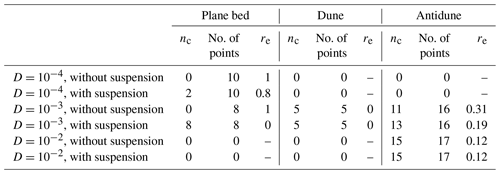As expected, most dune and antidune data plot in the unstable region, whereas several data points of dunes and antidunes plot in the stable region in both cases with and without suspension (Fig. 2, Table 1).

## 3.2D–Fr diagram

The contour maps of the maximum growth rate on the DFr plane also show that the stable region is larger in the diagram with suspension than in that without suspension (Fig. 3). For fine sediment, the upper limit of the stable region is smaller in the diagram with suspension than in that without suspension (Fig. 3a, b), whereas that does not much differ in the medium-sand case (Fig. 3c, d). Comparing with the observational data, most plane bed data plot in the stable region in the case of the stability analysis with suspension (Fig. 3). Also, most dune and antidune data plot in the unstable region in cases both with and without suspension (Fig. 3). The error rate for plane bed data decreased from 1 to 0.6 (Rep=5.62) and 0.45 to 0.18 (Rep=15.9) by adding the term of the suspended load (Table 2). For dunes and antidunes, the error rate does not differ between the cases with and without suspension, except for the antidune in the case of fine sediment, where re decreases from 0.6 to 0.2 (Table 2).

Table 2Error rates for the case of fixed Rep. The parameter nc denotes the number of correctly classified data points, and re is the error rate.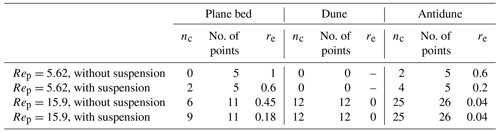4 Discussion

The role of suspended loads in the formation of plane beds and suppression of dune-scale instabilities is quantitatively illustrated as the broadening of the stable regions (Figs. 2 and 3). The stability diagrams show good agreement with the observational data of plane beds under flows with suspension. The transition from dunes to plane beds is explained by the spatial lag δ between the bed topography and the local sediment transport rate . If the bed topography and sediment transport rate are entirely in phase (δ=0), dunes migrate downstream without growth or decay. The dune height increases and decreases when the maximum sediment transport rate occurs upstream (δ<0) and downstream (δ>0) of the dune crest, respectively. introduced the spatial lag in his flow model to account for the bedform growth and decay, and subsequent research has investigated the effect of spatial lag on the bedform development . Recently, quantitatively observed the positive spatial lag under suspended-load-dominated flows in their flume experiments. Our analyses confirm that suspended loads dampen the development of bed waves, thereby facilitating the formation of plane beds, and thus cannot be neglected in theoretical analyses for realistic predictions of bedforms.

We found that dunes are deformed under flows with suspended loads, although further work is needed to investigate the amplitudes of dunes under such conditions. Field surveys have indicated the existence of low-angle dunes in suspended-load-dominated rivers ; moreover, flume experiments have indicated that dune height decreases with increasing suspended-load flux . Theoretical analyses in have also predicted a decrease in dune steepness under unsteady flows with suspension where the flow discharges were being increased. In future works, nonlinear analyses should be done to obtain the amplitudes of dunes under flows with suspended loads.

Ultimately, our linear analyses provide a possible explanation for the absence of dunes in turbidites: suspended loads suppress dune formation and facilitate plane bed formation. Previous research has suggested that the formation of dunes is suppressed due to the insufficient time for dune development (Walker1965), the hysteresis effect under waning flow conditions , the turbulence suppression by high suspended-sediment concentrations (Lowe1988), the lack of a sharp near-bed density gradient (Arnott2012), and the effect of clay-sized sediment on bed rheology . Although these interpretations could explain the absence of dune-scale cross-lamination in turbidites, we show that dune formation is suppressed without considering the above conditions. Although the above conditions may contribute to the deformation of dunes, instead, we propose that the development of dune-scale bed waves under turbidity currents is restricted by the presence of suspended loads. The model can be improved by the inclusion of such an effect in future studies.

5 Conclusions

We investigated the influence of suspended loads on the formation of plane beds under open-channel flows. The stability diagrams show that the stable region for finer sediments is wider in the diagram with suspension than that without suspension. Further, the published data of plane beds with suspension coincide well with the stability diagrams where the suspension was considered. Our theoretical analysis found that suspended loads promote the formation of plane beds and suppress the formation of dunes on the fine-grained bed. These results suggest that dune-scale cross-lamination is absent in turbidites because the development of dunes in turbidity currents is restricted by the presence of suspended loads. Additional theoretical work can be improved in future studies by the inclusion of possible mechanisms for the absence of dunes in turbidites.

Appendix A: Linear analysis

In Sect. 2.2.12.2.6, we formulate the hydrodynamics, the sediment transport model, and the basic state (Figs. A1 and A2). Here, we solve the equations obtained in the above sections.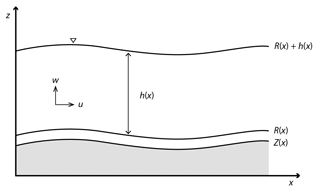Figure A1Conceptual diagram of the flow. The dimensionless parameters u and w are the flow velocities in the x and z directions, respectively; h is the flow depth; Z denotes the bed height; and R is the height of the reference level at which the flow velocity is assumed to vanish following a logarithmic law.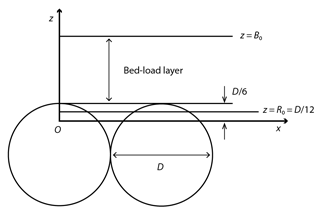Figure A2Conceptual diagram of the sediment bed. The origin of the z direction is denoted by O. The parameter D is the dimensionless diameter of a bed particle, B0 is the height of the top of the bed-load layer in the basic state, and R0 is the height of the reference level in the basic state.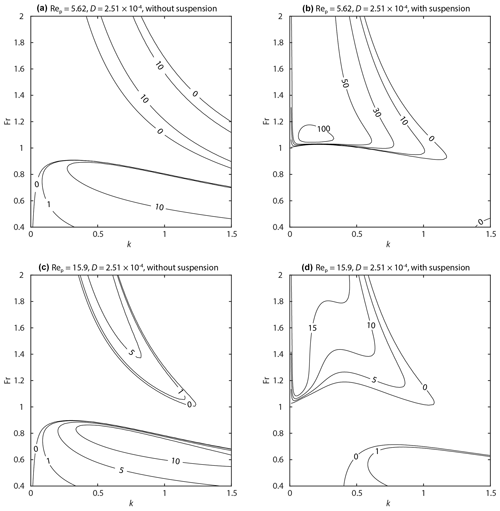Figure A3Contour map of perturbation growth rate ωi. The dimensionless particle diameter D was set to $D=\mathrm{2.51}×{\mathrm{10}}^{-\mathrm{4}}$. (a) Rep=5.62 without suspension. (b) Rep=5.62 with suspension. (c) Rep=15.9 without suspension. (d) Rep=15.9 with suspension.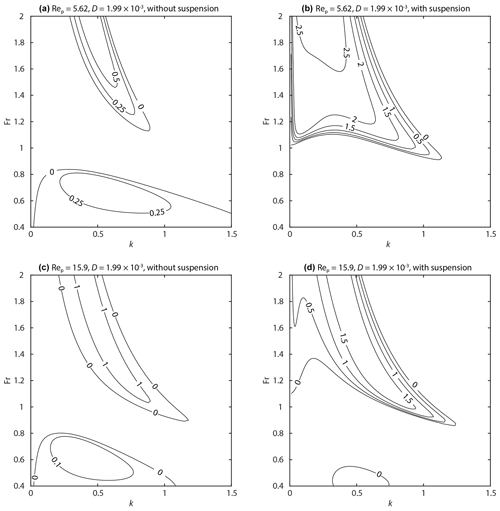Figure A4Contour map of perturbation growth rate ωi. The dimensionless particle diameter D was set to $D=\mathrm{1.99}×{\mathrm{10}}^{-\mathrm{3}}$. (a) Rep=5.62 without suspension. (b) Rep=5.62 with suspension. (c) Rep=15.9 without suspension. (d) Rep=15.9 with suspension.

Table A3Summary of data used for the stability diagram with $D={\mathrm{10}}^{-\mathrm{4}}$.Table A4Summary of data used for the stability diagram with $D={\mathrm{10}}^{-\mathrm{3}}$.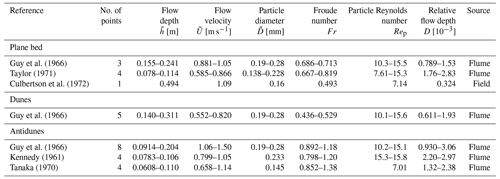Table A5Summary of data used for the stability diagram with $D={\mathrm{10}}^{-\mathrm{2}}$.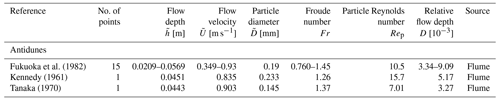We impose an infinitesimal perturbation on the basic state. All the variables are modified using a small amplitude A and a complex angular frequency of the perturbation ω as follows:

$\begin{array}{}\text{(A1)}& \begin{array}{rl}& \left(\mathit{\psi },p,h,Z,R,B,c\right)=\left({\mathit{\psi }}_{\mathrm{0}},{p}_{\mathrm{0}},\mathrm{1},\mathrm{0},{R}_{\mathrm{0}},{B}_{\mathrm{0}},{c}_{\mathrm{0}}\right)\\ & \phantom{\rule{0.25em}{0ex}}\phantom{\rule{0.25em}{0ex}}\phantom{\rule{0.25em}{0ex}}\phantom{\rule{0.25em}{0ex}}\phantom{\rule{0.25em}{0ex}}\phantom{\rule{0.25em}{0ex}}+A\left({\mathit{\psi }}_{\mathrm{1}},{p}_{\mathrm{1}},{H}_{\mathrm{1}},{Z}_{\mathrm{1}},{R}_{\mathrm{1}},{B}_{\mathrm{1}},{c}_{\mathrm{1}}\right)\mathrm{exp}\left[i\left(k\mathit{\xi }-\mathit{\omega }t\right)\right].\end{array}\end{array}$

The subscript 1 denotes a variable at 𝒪(A). By substituting Eq. (A1) into the governing equations and boundary conditions, we can obtain the following equations at 𝒪(A):

$\begin{array}{}\text{(A2)}& {\mathcal{L}}^{\mathit{\psi }}\left(\mathit{\eta }\right)\phantom{\rule{0.125em}{0ex}}{\mathit{\psi }}_{\mathrm{1}}\left(\mathit{\eta }\right)+{\mathcal{L}}^{h}\left(\mathit{\eta }\right)\phantom{\rule{0.125em}{0ex}}{H}_{\mathrm{1}}+{\mathcal{L}}^{R}\left(\mathit{\eta }\right)\phantom{\rule{0.125em}{0ex}}{R}_{\mathrm{1}}=\mathrm{0}\text{(A3)}& ik{p}_{\mathrm{1}}\left(\mathit{\eta }\right)+{\mathcal{P}}^{\mathit{\psi }}\left(\mathit{\eta }\right)\phantom{\rule{0.125em}{0ex}}{\mathit{\psi }}_{\mathrm{1}}\left(\mathit{\eta }\right)+{\mathcal{P}}^{h}\left(\mathit{\eta }\right)\phantom{\rule{0.125em}{0ex}}{H}_{\mathrm{1}}+{\mathcal{P}}^{R}\left(\mathit{\eta }\right)\phantom{\rule{0.125em}{0ex}}{R}_{\mathrm{1}}=\mathrm{0}.\end{array}$

Here, ϕ and ${\mathcal{P}}^{\mathit{\varphi }}\phantom{\rule{0.25em}{0ex}}\left(\mathit{\varphi }=\mathit{\psi },h,R\right)$ are linear operators. The specific forms of ϕ and 𝒫ϕ are skipped herein. With the use of the boundary conditions (Eqs. 35 and 36), we get

Additionally, Eqs. (A3) and (A5) give

$\begin{array}{}\text{(A8)}& {\mathcal{P}}^{\mathit{\psi }}\left(\mathrm{1}\right)\phantom{\rule{0.125em}{0ex}}{\mathit{\psi }}_{\mathrm{1}}\left(\mathrm{1}\right)+{\mathcal{P}}^{h}\left(\mathrm{1}\right)\phantom{\rule{0.125em}{0ex}}{H}_{\mathrm{1}}+{\mathcal{P}}^{R}\left(\mathrm{1}\right)\phantom{\rule{0.125em}{0ex}}{R}_{\mathrm{1}}=\mathrm{0}.\end{array}$

We employ a spectral collocation method using Chebyshev polynomials to solve the above differential equations. We expand ψ1 using the Chebyshev polynomials as follows:

$\begin{array}{}\text{(A9)}& {\mathit{\psi }}_{\mathrm{1}}=\sum _{n=\mathrm{0}}^{N}{a}_{n}{T}_{n}\left(\mathit{\zeta }\right),\end{array}$

where an is the coefficient for the nth-order Chebyshev polynomial Tn, and ζ is the independent variable of the Chebyshev polynomials defined in the domain [$-\mathrm{1},\mathrm{1}$]. In this study, we transform ζ using the following equation to improve the calculation accuracy:

$\begin{array}{}\text{(A10)}& \mathit{\zeta }=\mathrm{2}\left\{\frac{\mathrm{ln}\left[\left(\mathit{\eta }+{R}_{\mathrm{0}}\right)/{R}_{\mathrm{0}}\right]}{\mathrm{ln}\left[\left(\mathrm{1}+{R}_{\mathrm{0}}\right)/{R}_{\mathrm{0}}\right]}\right\}-\mathrm{1}.\end{array}$

The above functions are substituted into Eq. (A2), and then we evaluate the equation at the Gauss–Labatte points, which are defined as

$\begin{array}{}\text{(A11)}& {\mathit{\zeta }}_{j}=\mathrm{cos}\left(\frac{j\mathit{\pi }}{N+\mathrm{2}}\right),j=\mathrm{1},\mathrm{2},\mathrm{\dots },N+\mathrm{1}.\end{array}$

By combining the governing equations, boundary conditions, and closure assumptions, we obtain the following system of linear algebraic equations:

$\begin{array}{}\text{(A12)}& \mathbf{La}=\mathbf{M}{R}_{\mathrm{1}},\end{array}$

with

$\begin{array}{}\text{(A13)}& \mathbf{L}=\left(\begin{array}{cccc}{T}_{\mathrm{0}}\left(-\mathrm{1}\right)& \mathrm{\cdots }& {T}_{N}\left(-\mathrm{1}\right)& \mathrm{0}\\ \stackrel{\mathrm{ˇ}}{\mathcal{h}}\phantom{\rule{0.125em}{0ex}}{T}_{\mathrm{0}}\left(-\mathrm{1}\right)& \mathrm{\cdots }& \stackrel{\mathrm{ˇ}}{\mathcal{h}}\phantom{\rule{0.125em}{0ex}}{T}_{N}\left(-\mathrm{1}\right)& \mathrm{0}\\ {T}_{\mathrm{0}}\left(\mathrm{1}\right)& \mathrm{\cdots }& {T}_{N}\left(\mathrm{1}\right)& \mathrm{0}\\ {\stackrel{\mathrm{ˇ}}{\mathcal{P}}}^{\mathit{\psi }}\phantom{\rule{0.125em}{0ex}}{T}_{\mathrm{0}}\left(\mathrm{1}\right)& \mathrm{\cdots }& {\stackrel{\mathrm{ˇ}}{\mathcal{P}}}^{\mathit{\psi }}\phantom{\rule{0.125em}{0ex}}{T}_{N}\left(\mathrm{1}\right)& {\stackrel{\mathrm{ˇ}}{\mathcal{P}}}^{h}\\ {\stackrel{\mathrm{ˇ}}{\mathcal{L}}}^{\mathit{\psi }}\phantom{\rule{0.125em}{0ex}}{T}_{\mathrm{0}}\left({\mathit{\zeta }}_{\mathrm{2}}\right)& \mathrm{\cdots }& {\stackrel{\mathrm{ˇ}}{\mathcal{L}}}^{\mathit{\psi }}\phantom{\rule{0.125em}{0ex}}{T}_{N}\left({\mathit{\zeta }}_{\mathrm{2}}\right)& {\stackrel{\mathrm{ˇ}}{\mathcal{L}}}^{h}\\ \mathrm{⋮}& \mathrm{\ddots }& \mathrm{⋮}& \mathrm{⋮}\\ {\stackrel{\mathrm{ˇ}}{\mathcal{L}}}^{\mathit{\psi }}\phantom{\rule{0.125em}{0ex}}{T}_{\mathrm{0}}\left({\mathit{\zeta }}_{N-\mathrm{2}}\right)& \mathrm{\cdots }& {\stackrel{\mathrm{ˇ}}{\mathcal{L}}}^{\mathit{\psi }}\phantom{\rule{0.125em}{0ex}}{T}_{N}\left({\mathit{\zeta }}_{N-\mathrm{2}}\right)& {\stackrel{\mathrm{ˇ}}{\mathcal{L}}}^{h}\end{array}\right)\text{(A14)}& \mathbf{a}=\left({a}_{\mathrm{0}},{a}_{\mathrm{1}},\mathrm{\dots },{a}_{N},{D}_{\mathrm{1}}\right)\text{(A15)}& \mathbf{M}=\left(\mathrm{0},\mathrm{0},\mathrm{0},{\stackrel{\mathrm{ˇ}}{\mathcal{P}}}^{R},{\stackrel{\mathrm{ˇ}}{\mathcal{L}}}^{h},\mathrm{\dots },{\stackrel{\mathrm{ˇ}}{\mathcal{L}}}^{h}\right),\end{array}$

where a check mark denotes a linear operator associated with variable transformation from η to ζ. We obtain the following solution from Eq. (A12):

$\begin{array}{}\text{(A16)}& \mathbf{a}={\mathbf{L}}^{-\mathrm{1}}\mathbf{M}{R}_{\mathrm{1}}.\end{array}$

Additionally, Eqs. (A9) and (A16) give

$\begin{array}{}\text{(A17)}& {\mathit{\psi }}_{\mathrm{1}}={\mathit{\psi }}_{\mathrm{1}}^{*}\left(\mathit{\eta }\right){R}_{\mathrm{1}}\text{(A18)}& {H}_{\mathrm{1}}={H}_{\mathrm{1}}^{*}{R}_{\mathrm{1}}.\end{array}$

Similarly, we solve the eigenvalue problems for the sediment transport equations. By substituting Eq. (A1) into Eq. (24), we obtain the following equations of the order of 𝒪(A):

$\begin{array}{}\text{(A19)}& {\mathcal{C}}^{c}{c}_{\mathrm{1}}\left(\mathit{\eta }\right)+{\mathcal{C}}^{\mathit{\psi }}\left(\mathit{\eta }\right)\phantom{\rule{0.125em}{0ex}}{\mathit{\psi }}_{\mathrm{1}}\left(\mathit{\eta }\right)+{\mathcal{C}}^{H}{H}_{\mathrm{1}}+{\mathcal{C}}^{R}{R}_{\mathrm{1}}=\mathrm{0}.\end{array}$

Based on Eqs. (A17) and (A18), we obtain

$\begin{array}{}\text{(A20)}& {\mathcal{C}}^{c}{c}_{\mathrm{1}}\left(\mathit{\eta }\right)+\left({\mathcal{C}}^{\mathit{\psi }}\left(\mathit{\eta }\right)\phantom{\rule{0.125em}{0ex}}{\mathit{\psi }}_{\mathrm{1}}^{*}\left(\mathit{\eta }\right)+{\mathcal{C}}^{H}{H}_{\mathrm{1}}^{*}+{\mathcal{C}}^{R}\right){R}_{\mathrm{1}}=\mathrm{0}.\end{array}$

The boundary conditions give

$\begin{array}{}\text{(A21)}& {\mathcal{S}}^{c}{c}_{\mathrm{1}}\left(\mathrm{1}\right)+\left({\mathcal{S}}^{\mathit{\psi }}\left(\mathrm{1}\right)\phantom{\rule{0.125em}{0ex}}{\mathit{\psi }}_{\mathrm{1}}^{*}\left(\mathrm{1}\right)+{\mathcal{S}}^{H}{H}_{\mathrm{1}}^{*}+{\mathcal{S}}^{R}\right){R}_{\mathrm{1}}=\mathrm{0}\text{(A22)}& {\mathcal{B}}^{c}{c}_{\mathrm{1}}\left(\mathrm{0}\right)+\left({\mathcal{B}}^{\mathit{\psi }}\left(\mathrm{0}\right)\phantom{\rule{0.125em}{0ex}}{\mathit{\psi }}_{\mathrm{1}}^{*}\left(\mathrm{0}\right)+{\mathcal{B}}^{H}{H}_{\mathrm{1}}^{*}+{\mathcal{B}}^{R}\right){R}_{\mathrm{1}}=\mathrm{0}.\end{array}$

Here, 𝒞ϕ, 𝒮ϕ, and ${\mathcal{B}}^{\mathit{\varphi }}\phantom{\rule{0.25em}{0ex}}\left(\mathit{\varphi }=\mathit{\psi },h,R,c\right)$ are the linear operators.

We expand c1 using Chebyshev polynomials as follows:

$\begin{array}{}\text{(A23)}& {c}_{\mathrm{1}}=\sum _{n=\mathrm{0}}^{N}{b}_{n}{T}_{n}\left(\mathit{\zeta }\right).\end{array}$

The system is evaluated at the Gauss–Labatte points, and then we obtain

$\begin{array}{}\text{(A24)}& \mathbf{Kb}=\mathbf{N}{R}_{\mathrm{1}},\end{array}$

with

$\begin{array}{}\text{(A25)}& \mathbf{K}=\left(\begin{array}{ccc}{\stackrel{\mathrm{ˇ}}{\mathcal{B}}}^{c}{T}_{\mathrm{0}}\left(-\mathrm{1}\right)& \mathrm{\cdots }& {\stackrel{\mathrm{ˇ}}{\mathcal{B}}}^{c}{T}_{N}\left(-\mathrm{1}\right)\\ {\stackrel{\mathrm{ˇ}}{\mathcal{S}}}^{c}{T}_{\mathrm{0}}\left(\mathrm{1}\right)& \mathrm{\cdots }& {\stackrel{\mathrm{ˇ}}{\mathcal{S}}}^{c}{T}_{N}\left(-\mathrm{1}\right)\\ {\stackrel{\mathrm{ˇ}}{\mathcal{C}}}^{c}{T}_{\mathrm{0}}\left({\mathit{\zeta }}_{\mathrm{1}}\right)& \mathrm{\cdots }& {\stackrel{\mathrm{ˇ}}{\mathcal{C}}}^{c}{T}_{N}\left({\mathit{\zeta }}_{\mathrm{1}}\right)\\ \mathrm{⋮}& \mathrm{\ddots }& \mathrm{⋮}\\ {\stackrel{\mathrm{ˇ}}{\mathcal{C}}}^{c}{T}_{\mathrm{0}}\left({\mathit{\zeta }}_{N-\mathrm{1}}\right)& \mathrm{\cdots }& {\stackrel{\mathrm{ˇ}}{\mathcal{C}}}^{c}{T}_{N}\left({\mathit{\zeta }}_{N-\mathrm{1}}\right)\end{array}\right)\end{array}$
$\begin{array}{}\text{(A26)}& \mathbf{b}=\left({b}_{\mathrm{0}},{b}_{\mathrm{1}},\mathrm{\dots },{b}_{N}\right)\text{(A27)}& \mathbf{N}=-\left(\begin{array}{c}{\stackrel{\mathrm{ˇ}}{\mathcal{B}}}^{\mathit{\psi }}{\mathit{\psi }}_{\mathrm{1}}^{*}\left(-\mathrm{1}\right)+{\stackrel{\mathrm{ˇ}}{\mathcal{B}}}^{h}{H}_{\mathrm{1}}^{*}+{\stackrel{\mathrm{ˇ}}{\mathcal{B}}}^{R}\\ {\stackrel{\mathrm{ˇ}}{\mathcal{S}}}^{\mathit{\psi }}{\mathit{\psi }}_{\mathrm{1}}^{*}\left(\mathrm{1}\right)+{\stackrel{\mathrm{ˇ}}{\mathcal{S}}}^{h}{H}_{\mathrm{1}}^{*}+{\stackrel{\mathrm{ˇ}}{\mathcal{S}}}^{R}\\ {\stackrel{\mathrm{ˇ}}{\mathcal{C}}}^{\mathit{\psi }}{\mathit{\psi }}_{\mathrm{1}}^{*}\left({\mathit{\zeta }}_{\mathrm{1}}\right)+{\stackrel{\mathrm{ˇ}}{\mathcal{C}}}^{h}{H}_{\mathrm{1}}^{*}+{\stackrel{\mathrm{ˇ}}{\mathcal{C}}}^{R}\\ \mathrm{⋮}\\ {\stackrel{\mathrm{ˇ}}{\mathcal{C}}}^{\mathit{\psi }}{\mathit{\psi }}_{\mathrm{1}}^{*}\left({\mathit{\zeta }}_{N-\mathrm{1}}\right)+{\stackrel{\mathrm{ˇ}}{\mathcal{C}}}^{h}{H}_{\mathrm{1}}^{*}+{\stackrel{\mathrm{ˇ}}{\mathcal{C}}}^{R}\end{array}\right).\end{array}$

The coefficient bn is derived as

$\begin{array}{}\text{(A28)}& \mathbf{b}={\mathbf{K}}^{-\mathrm{1}}\mathbf{N}{R}_{\mathrm{1}}.\end{array}$

Therefore, the following equation is obtained:

$\begin{array}{}\text{(A29)}& {c}_{\mathrm{1}}\left(\mathit{\eta }\right)={c}_{\mathrm{1}}^{*}\left(\mathit{\eta }\right){R}_{\mathrm{1}}.\end{array}$

By substituting Eqs. (A17), (A18), and (A29) into Exner's equation (Eq. 61), the complex angular frequency ω is obtained in the following form:

$\begin{array}{}\text{(A30)}& \mathit{\omega }=\mathit{\omega }\left(k,Fr,{C}_{z},R{e}_{\mathrm{p}}\right)={\mathit{\omega }}_{\mathrm{r}}+i{\mathit{\omega }}_{i},\end{array}$

where ωi corresponds to the growth rate of the perturbation.

Here, using $R{e}_{\mathrm{p}}=R{e}_{\mathrm{p}}\left(D\right)=R{e}_{\mathrm{p}}\left(\stackrel{\mathrm{̃}}{D},{\stackrel{\mathrm{̃}}{h}}_{\mathrm{0}}\right)$ (Eq. 27) and ${C}_{z}={C}_{z}\left({R}_{\mathrm{0}}\right)={C}_{z}\left(\stackrel{\mathrm{̃}}{D},{\stackrel{\mathrm{̃}}{h}}_{\mathrm{0}}\right)$ (Eq. 52), we can rewrite Eq. (A30) as

$\begin{array}{}\text{(A31)}& \mathit{\omega }=\mathit{\omega }\left(k,Fr,\stackrel{\mathrm{̃}}{D},{\stackrel{\mathrm{̃}}{h}}_{\mathrm{0}}\right).\end{array}$

Thus, we can obtain the growth rate ωi as a function of k for a given combination of Fr, $\stackrel{\mathrm{̃}}{D}$, and ${\stackrel{\mathrm{̃}}{h}}_{\mathrm{0}}$.

Code and data availability

The datasets and codes used for this study can be found at https://doi.org/10.5281/zenodo.8332448 (Ohata et al., 2023). Unpublished data used for the analysis were cited from the dataset of (https://doi.org/10.22002/d1.943).

Author contributions

KO and NI performed the linear-stability analysis. HN and NI contributed to the interpretation of the results. KO wrote the manuscript and prepared the figures, and then HN and NI provided feedback on the manuscript and figures.

Competing interests

The contact author has declared that none of the authors has any competing interests.

Disclaimer

Publisher’s note: Copernicus Publications remains neutral with regard to jurisdictional claims in published maps and institutional affiliations.

Acknowledgements

We would like to express our gratitude to Robert Dorrell for his comments. We are thankful to the anonymous referees for their insightful comments on earlier versions of the manuscript.

Financial support

This research has been supported by the Japan Society for the Promotion of Science (grant no.  18J22211 and 20H01985).

Review statement

This paper was edited by Daniel Parsons and reviewed by three anonymous referees.

References

Arnott, R. W. C.: Turbidites, and the Case of the Missing Dunes, J. Sediment. Res., 82, 379–384, https://doi.org/10.2110/jsr.2012.29, 2012. a, b

Bohorquez, P., Cañada-Pereira, P., Jimenez-Ruiz, P. J., and del Moral-Erencia, J. D.: The fascination of a shallow-water theory for the formation of megaflood-scale dunes and antidunes, Earth-Sci. Rev., 193, 91–108, https://doi.org/10.1016/j.earscirev.2019.03.021, 2019. a, b, c

Bose, S. K. and Dey, S.: Reynolds averaged theory of turbulent shear flows over undulating beds and formation of sand waves, Phys. Rev. E, 80, 036304, https://doi.org/10.1103/PhysRevE.80.036304, 2009. a

Bouma, A. H.: Sedimentology of some flysch deposits: A graphic approach to facies interpretation, Elsevier Scientific Publishing Company, Amsterdam, 1962. a

Bourke, M. C., Lancaster, N., Fenton, L. K., Parteli, E. J. R., Zimbelman, J. R., and Radebaugh, J.: Extraterrestrial dunes: An introduction to the special issue on planetary dune systems, Geomorphology, 121, 1–14, https://doi.org/10.1016/j.geomorph.2010.04.007, 2010. a

Bradley, R., Venditti, J. G., Kostaschuk, R. A., Church, M., Hendershot, M., and Allison, M. A.: Flow and sediment suspension events over low-angle dunes: Fraser Estuary, Canada, J. Geophys. Res.-Earth, 118, 1693–1709, https://doi.org/10.1002/jgrf.20118, 2013. a

Bradley, R. W. and Venditti, J. G.: Transport scaling of dune dimensions in shallow flows, J. Geophys. Res.-Earth, 124, 526–547, https://doi.org/10.1029/2018JF004832, 2019. a

Bridge, J. S. and Best, J. L.: Flow, sediment transport and bedform dynamics over the transition from dunes to upper-stage plane beds: implications for the formation of planar laminae, Sedimentology, 35, 753–763, https://doi.org/10.1111/j.1365-3091.1988.tb01249.x, 1988. a, b

Brownlie, W. R.: Prediction of flow depth and sediment discharge in open channels, Tech. Rep. KH-R43A, W. M. Keck Laboratory of Hydraulics and Water Resources, California Institute of Technology, Pasadena, California, https://doi.org/10.7907/Z9KP803R, 1981. a

Brownlie, W. R.: Digitized dataset from “Compilation of alluvial channel data: laboratory and field” (Version 1.0), caltechDATA [data set], https://doi.org/10.22002/d1.943, 2018. a

Cisneros, J., Best, J., van Dijk, T., de Almeida, R. P., Amsler, M., Boldt, J., Freitas, B., Galeazzi, C., Huizinga, R., Ianniruberto, M., Ma, H., Nittrouer, J. A., Oberg, K., Orfeo, O., Parsons, D., Szupiany, R., Wang, P., and Zhang, Y.: Dunes in the world's big rivers are characterized by low-angle lee-side slopes and a complex shape, Nat. Geosci., 13, 156–162, https://doi.org/10.1038/s41561-019-0511-7, 2020. a

Colombini, M.: Revisiting the linear theory of sand dune formation, J. Fluid Mech., 502, 1–16, https://doi.org/10.1017/S0022112003007201, 2004. a, b, c, d, e

Colombini, M. and Stocchino, A.: Finite-amplitude river dunes, J. Fluid Mech., 611, 283–306, https://doi.org/10.1017/S0022112008002814, 2008. a, b

Culbertson, J. K., Scott, C. H., and Bennett, J. P.: Summary of alluvial-channel data from Rio Grande conveyance channel, New Mexico, 1965–69, Tech. Rep. 562-J, Professional Paper, Washington, DC, https://doi.org/10.3133/pp562J, 1972. a, b, c

de Almeida, R. P., Galeazzi, C. P., Freitas, B. T., Janikian, L., Ianniruberto, M., and Marconato, A.: Large barchanoid dunes in the Amazon River and the rock record: Implications for interpreting large river systems, Earth Planet. Sc. Lett., 454, 92–102, https://doi.org/10.1016/j.epsl.2016.08.029, 2016. a

de Leeuw, J., Lamb, M. P., Parker, G., Moodie, A. J., Haught, D., Venditti, J. G., and Nittrouer, J. A.: Entrainment and suspension of sand and gravel, Earth Surf. Dynam., 8, 485–504, https://doi.org/10.5194/esurf-8-485-2020, 2020. a, b

Di Cristo, C., Iervolino, M., and Vacca, A.: Linear stability analysis of a 1-D model with dynamical description of bed-load transport, J. Hydraul. Res., 44, 480–487, https://doi.org/10.1080/00221686.2006.9521699, 2006. a

Dietrich, W. E. and Whiting, P.: Boundary shear stress and sediment transport in river meanders of sand and gravel, Chap. 1, American Geophysical Union (AGU), 1–50, https://agupubs.onlinelibrary.wiley.com/doi/abs/10.1029/WM012p0001 (last access: 29 September 2023), 1989. a

Endo, N. and Masuda, F.: Small ripples in dunes regime and interpretation about dunes-missing in turbidite, J. Geol. Soc. Jpn., 103, 741–746, https://doi.org/10.5575/geosoc.103.741, 1997. a

Engelund, F.: Instability of erodible beds, J. Fluid Mech., 42, 225–244, https://doi.org/10.1017/S0022112070001210, 1970. a

Ferguson, R. and Church, M.: A simple universal equation for grain settling velocity, J. Sediment. Res., 74, 933–937, https://doi.org/10.1306/051204740933, 2004. a

Foley, M. G.: Scour and fill in ephemeral streams, Tech. Rep. KH-R:KH-R-33, W. M. Keck Laboratory of Hydraulics and Water Resources, California Institute of Technology, Pasadena, California, https://doi.org/10.7907/gbn5-jb14, 1975. a

Fourrière, A., Claudin, P., and Andreotti, B.: Bedforms in a turbulent stream: formation of ripples by primary linear instability and of dunes by nonlinear pattern coarsening, J. Fluid Mech., 649, 287–328, https://doi.org/10.1017/S0022112009993466, 2010. a

Fredsøe, J.: On the development of dunes in erodible channels, J. Fluid Mech., 64, 1–16, https://doi.org/10.1017/S0022112074001960, 1974. a

Fredsøe, J.: Unsteady flow in straight alluvial streams. Part 2. Transition from dunes to plane bed, J. Fluid Mech., 102, 431–453, https://doi.org/10.1017/S0022112081002723, 1981. a

Fukuoka, S., Okutsu, K., and Yamasaka, M.: Dynamic and kinematic features of sand waves in upper regime, P. Jpn. Soc. Civil Eng., 323, 77–89, https://doi.org/10.2208/jscej1969.1982.323_77, 1982 (in Japanese). a

Gao, X., Narteau, C., and Rozier, O.: Development and steady states of transverse dunes: A numerical analysis of dune pattern coarsening and giant dunes, J. Geophys. Res.-Earth, 120, 2200–2219, https://doi.org/10.1002/2015JF003549, 2015. a

Guy, H. P., Simons, D. B., and Richardson, E. V.: Summary of alluvial channel data from flume experiments, 1956–61, Tech. Rep. 462-I, Professional Paper, Washington, DC, https://doi.org/10.3133/pp462I, 1966. a, b, c, d, e, f

Hage, S., Cartigny, M. J. B., Clare, M. A., Sumner, E. J., Vendettuoli, D., Hughes Clarke, J. E., Hubbard, S. M., Talling, P. J., Lintern, D. G., Stacey, C. D., Englert, R. G., Vardy, M. E., Hunt, J. E., Yokokawa, M., Parsons, D. R., Hizzett, J. L., Azpiroz-Zabala, M., and Vellinga, A. J.: How to recognize crescentic bedforms formed by supercritical turbidity currents in the geologic record: Insights from active submarine channels, Geology, 46, 563–566, https://doi.org/10.1130/G40095.1, 2018. a

Harms, J. C.: Primary Sedimentary Structures, Annu. Rev. Earth Pl. Sc., 7, 227–248, https://doi.org/10.1146/annurev.ea.07.050179.001303, 1979. a

Hendershot, M. L., Venditti, J. G., Bradley, R. W., Kostaschuk, R. A., Church, M., and Allison, M. A.: Response of low-angle dunes to variable flow, Sedimentology, 63, 743–760, https://doi.org/10.1111/sed.12236, 2016. a

Kennedy, J. F.: Stationary waves and antidunes in alluvial channels, Tech. Rep. KH-R-2, W. M. Keck Laboratory of Hydraulics and Water Resources, California Institute of Technology, Pasadena, CA, https://doi.org/10.7907/Z9QR4V22, 1961. a, b, c

Kennedy, J. F.: The mechanics of dunes and antidunes in erodible-bed channels, J. Fluid Mech., 16, 521–544, https://doi.org/10.1017/S0022112063000975, 1963. a, b

Kostaschuk, R. and Villard, P.: Flow and sediment transport over large subaqueous dunes: Fraser River, Canada, Sedimentology, 43, 849–863, https://doi.org/10.1111/j.1365-3091.1996.tb01506.x, 1996. a, b

Lowe, D. R.: Suspended-load fallout rate as an independent variable in the analysis of current structures, Sedimentology, 35, 765–776, https://doi.org/10.1111/j.1365-3091.1988.tb01250.x, 1988. a, b

Ma, H., Nittrouer, J. A., Naito, K., Fu, X., Zhang, Y., Moodie, A. J., Wang, Y., Wu, B., and Parker, G.: The exceptional sediment load of fine-grained dispersal systems: Example of the Yellow River, China, Sci. Adv., 3, https://doi.org/10.1126/sciadv.1603114, 2017. a

McLean, S. R.: The stability of ripples and dunes, Earth-Sci. Rev., 29, 131–144, https://doi.org/10.1016/0012-8252(0)90032-Q, 1990. a

Miall, A.: Alluvial deposits, in: Facies models 4, edited by: James, N. P. and Dalrymple, R. W., Geological Association of Canada, Canada, 105–137, ISBN 9781897095508, 2010. a

Nakasato, Y. and Izumi, N.: Linear stability analysis of small-scale fluvial bed waves with active suspended sediment load, J. Appl. Mech., 11, 727–734, https://doi.org/10.2208/journalam.11.727, 2008 (in Japanese). a, b

Naqshband, S., Ribberink, J. S., Hurther, D., and Hulscher, S. J. M. H.: Bed load and suspended load contributions to migrating sand dunes in equilibrium, J. Geophys. Res.-Earth, 119, 1043–1063, https://doi.org/10.1002/2013JF003043, 2014. a, b

Naqshband, S., Hoitink, A. J. F., McElroy, B., Hurther, D., and Hulscher, S. J. M. H.: A sharp view on river dune transition to upper stage plane bed, Geophys. Res. Lett., 44, 11437–11444, https://doi.org/10.1002/2017GL075906, 2017. a, b, c

Ohata, K., Naruse, H., and Izumi, N.: Linear-stability analysis of plane beds under flows with suspended loads, Zenodo [data set and code], https://doi.org/10.5281/zenodo.8332448, 2023.

Sambrook Smith, G. H., Best, J. L., Leroy, J. Z., and Orfeo, O.: The alluvial architecture of a suspended sediment dominated meandering river: the Río Bermejo, Argentina, Sedimentology, 63, 1187–1208, https://doi.org/10.1111/sed.12256, 2016. a

Schindler, R. J., Parsons, D. R., Ye, L., Hope, J. A., Baas, J. H., Peakall, J., Manning, A. J., Aspden, R. J., Malarkey, J., Simmons, S., Paterson, D. M., Lichtman, I. D., Davies, A. G., Thorne, P. D., and Bass, S. J.: Sticky stuff: Redefining bedform prediction in modern and ancient environments, Geology, 43, 399–402, https://doi.org/10.1130/G36262.1, 2015. a, b

Smith, J. D. and McLean, S. R.: Spatially averaged flow over a wavy surface, J. Geophys. Res., 82, 1735–1746, https://doi.org/10.1029/JC082i012p01735, 1977. a, b

Talling, P. J., Masson, D. G., Sumner, E. J., and Malgesini, G.: Subaqueous sediment density flows: Depositional processes and deposit types, Sedimentology, 59, 1937–2003, https://doi.org/10.1111/j.1365-3091.2012.01353.x, 2012. a

Tanaka, Y.: An experimental study on anti-dunes, Disaster Prev. Res. Inst. Annu., 13, 271–284, 1970. a, b, c

Taylor, B. D.: Temperature effects in alluvial streams, Tech. Rep. KH-R-27, W. M. Keck Laboratory of Hydraulics and Water Resources, California Institute of Technology, Pasadena, CA, https://doi.org/10.7907/Z93776PN, 1971. a, b

Tilston, M., Arnott, R., Rennie, C., and Long, B.: The influence of grain size on the velocity and sediment concentration profiles and depositional record of turbidity currents, Geology, 43, 839–842, https://doi.org/10.1130/G37069.1, 2015. a

van den Berg, J. H. and van Gelder, A.: A new bedform stability diagram, with emphasis on the transition of ripples to plane bed in flows over fine sand and silt, in: Alluvial sedimentation, edited by: Marzo, M. and Puigdefabregas, C., Vol. 17, Blackwell Scientific Publications, Special Publications, International Association of Sedimentologists, 11–21, https://doi.org/10.1002/9781444303995.ch2, 1993. a

van Duin, O. J. M., Hulscher, S. J. M. H., Ribberink, J. S., and Dohmen-Janssen, C. M.: Modeling of spatial lag in bed-load transport processes and its effect on dune morphology, J. Hydraul. Eng., 143, 04016084, https://doi.org/10.1061/(ASCE)HY.1943-7900.0001254, 2017. a, b

Vesipa, R., Camporeale, C., and Ridolfi, L.: A shallow-water theory of river bedforms in supercritical conditions, Phys. Fluids, 24, 94104, https://doi.org/10.1063/1.4753943, 2012. a

Walker, R. G.: The origin and significance of the internal sedimentay structures of turbidites, P. Yorks. Geol. Soc., 35, 1–32, https://doi.org/10.1144/pygs.35.1.1, 1965. a

Wong, M. and Parker, G.: Reanalysis and Correction of Bed-Load Relation of Meyer-Peter and Müller Using Their Own Database, J. Hydraul. Eng., 132, 1159–1168, https://doi.org/10.1061/(ASCE)0733-9429(2006)132:11(1159), 2006. a, b

Yokokawa, M., Izumi, N., Naito, K., Parker, G., Yamada, T., and Greve, R.: Cyclic steps on ice, J. Geophys. Res.-Earth, 121, 1023–1048, https://doi.org/10.1002/2015JF003736, 2016. a